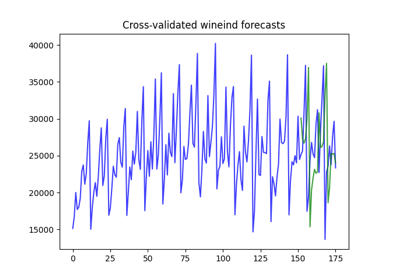# pmdarima.model_selection.SlidingWindowForecastCV¶

class pmdarima.model_selection.SlidingWindowForecastCV(h=1, step=1, window_size=None)[source][source]

Use a sliding window to perform cross validation

This approach to CV slides a window over the training samples while using several future samples as a test set. While similar to the RollingForecastCV, it differs in that the train set does not grow, but rather shifts.

Parameters: h : int, optional (default=1) The forecasting horizon, or the number of steps into the future after the last training sample for the test set. step : int, optional (default=1) The size of step taken between training folds. window_size : int or None, optional (default=None) The size of the rolling window to use. If None, a rolling window of size n_samples // 5 will be used.

Attributes

 horizon The forecast horizon for the cross-validator

References

Examples

With a step size of one and a forecasting horizon of one, the training size will grow by 1 for each step, and the test index will be 1 + the last training index. Notice the sliding window also adjusts where the training sample begins for each fold:

>>> import pmdarima as pm
>>> from pmdarima.model_selection import SlidingWindowForecastCV
>>> cv = SlidingWindowForecastCV()
>>> cv_generator = cv.split(wineind)
>>> next(cv_generator)
(array([ 0,  1,  2,  3,  4,  5,  6,  7,  8,  9, 10, 11, 12, 13, 14, 15, 16,
17, 18, 19, 20, 21, 22, 23, 24, 25, 26, 27, 28, 29, 30, 31, 32, 33,
34]), array())
>>> next(cv_generator)
(array([ 1,  2,  3,  4,  5,  6,  7,  8,  9, 10, 11, 12, 13, 14, 15, 16, 17,
18, 19, 20, 21, 22, 23, 24, 25, 26, 27, 28, 29, 30, 31, 32, 33, 34,
35]), array())


With a step size of 4, a forecasting horizon of 6, and a window size of 12, the training size will grow by 4 for each step, and the test index will 6 + the last index in the training fold:

>>> cv = SlidingWindowForecastCV(step=4, h=6, window_size=12)
>>> cv_generator = cv.split(wineind)
>>> next(cv_generator)
(array([ 0,  1,  2,  3,  4,  5,  6,  7,  8,  9, 10, 11]),
array([12, 13, 14, 15, 16, 17]))
>>> next(cv_generator)
(array([ 4,  5,  6,  7,  8,  9, 10, 11, 12, 13, 14, 15]),
array([16, 17, 18, 19, 20, 21]))


Methods

 get_params([deep]) Get parameters for this estimator. set_params(**params) Set the parameters of this estimator. split(y[, exogenous]) Generate indices to split data into training and test sets
__init__(h=1, step=1, window_size=None)[source][source]

Initialize self. See help(type(self)) for accurate signature.

get_params(deep=True)[source]

Get parameters for this estimator.

Parameters: deep : bool, default=True If True, will return the parameters for this estimator and contained subobjects that are estimators. params : mapping of string to any Parameter names mapped to their values.
horizon

The forecast horizon for the cross-validator

set_params(**params)[source]

Set the parameters of this estimator.

The method works on simple estimators as well as on nested objects (such as pipelines). The latter have parameters of the form <component>__<parameter> so that it’s possible to update each component of a nested object.

Parameters: **params : dict Estimator parameters. self : object Estimator instance.
split(y, exogenous=None)[source]

Generate indices to split data into training and test sets

Parameters: y : array-like or iterable, shape=(n_samples,) The time-series array. exogenous : array-like, shape=[n_obs, n_vars], optional (default=None) An optional 2-d array of exogenous variables. train : np.ndarray The training set indices for the split test : np.ndarray The test set indices for the split

## Examples using pmdarima.model_selection.SlidingWindowForecastCV¶Cross-validation predictions